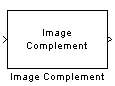# Image Complement

Compute the complement of pixel values in binary or intensity images

• Library:
• Computer Vision Toolbox / Conversions

•## Description

The Image Complement block computes the complement of a binary or intensity image.

For binary images, the block replaces pixel values equal to `0` with `1` and pixel values equal to `1` with `0`. In the output image, black and white are reversed. For intensity images, the block subtracts each pixel value from the maximum value that can be represented by the input data type and outputs the difference. In the output image, dark areas become lighter and light areas become darker.

For example, suppose the input pixel values are given by x(i) and the output pixel values are given by y(i). If the data type of the input is boolean, double or single, the block outputs y(i) = 1.0-x(i). If the input is an 8-bit unsigned integer, the block outputs y(i) = 255-x(i).

## Ports

### Input

expand all

Specify an input image as a matrix of intensity values.

Data Types: `single` | `double` | `int8` | `int16` | `int32` | `int64` | `uint8` | `uint16` | `uint32` | `uint64` | `Boolean`

### Output

expand all

The complement of the binary or intensity input image is returned as a matrix of intensity values. The size and data type of the output image are the same as those of the input image.

Data Types: `single` | `double` | `int8` | `int16` | `int32` | `int64` | `uint8` | `uint16` | `uint32` | `uint64` | `Boolean`

## Block Characteristics

 Data Types `Boolean` | `double` | `integer` | `single` Multidimensional Signals `no` Variable-Size Signals `yes`

## Version History

Introduced before R2006a